Home > A2C > Chapter 8 > Lesson 8.1.7 > Problem8-107

8-107.
1. Convert each of the following angle measures. Give exact answers. Homework Help ✎

1.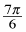radians to degrees

2.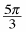radians to degrees

3. 45 degrees to radians

4. 100° to radians

5. 810° to radians

6.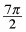radians to degrees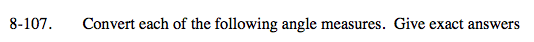There are multiple ways to approach these problems. Try different strategies to expand your personal toolkit.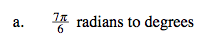Use Hint 1 from
problem 8-106.
Let π = 180° and simplify.
Notice, you do not need a calculator if you divide 180 by 6 as your first step.

210°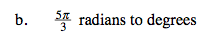Use Hint 1 or 2 from 8-106.

$\text{Multiply } \frac{5 \pi}{3} \text{ by } \frac{180}{\pi}.$

Simplify by factoring out Giant Ones.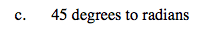Use the second part of Hint 2 from 8-106.

$\frac{{\pi}}{4}$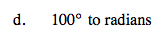Use Hint 2 from 8-106 or try Hint 3:

$\text{Use the proportion } \frac{\pi}{180°} = \frac{r}{100°}.$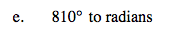See parts (c) and (d).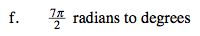See parts (a) and (b).

630°